Friday, June 9, 2023
HomeAnalog to Digital Converter (ADC)Analog to Digital Converter (ADC) - How it Works, Types, Applications

# Analog to Digital Converter (ADC) – How it Works, Types, Applications

Analog to Digital Converter (ADC) is an integrated circuit which helps to transform analog signal to digital form. This post will discuss about what is an Analog to Digital Converter (ADC), its various types, how it works, applications, advantages and disadvantages.

## What is Analog to Digital Converter (ADC)

Analog to Digital Converter (ADC) basically converts physical variables which are analog in nature to digital signal for processing. They have high conversion efficiency and requires less power. Examples of physical variables include audio signals, temperature, pressure etc.Fig. 1 – Introduction to Analog to Digital Converter

It can also be defined as a device that provides a digital output which is the representation of the input voltage or current level.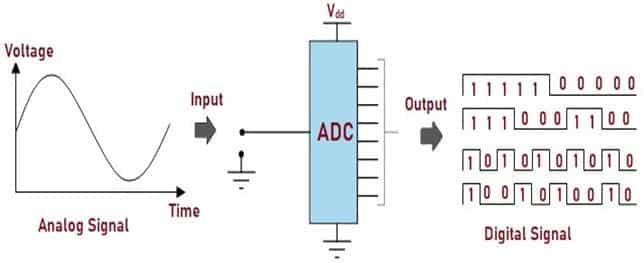Fig. 2 – Schematic Diagram of ADC

## Types of Analog to Digital Converter

The different types of Analog to Digital Converters (ADCs) are:

The operation of Flash ADC is the fastest compared to all other ADCs. Hence, Flash ADCs are used in the applications which requires High-speed and large bandwidth. It is also known as Parallel ADC.

Fig.3 below illustrates block diagram of Analog to Digital Converter (ADC) where Analog input is connected to all comparators so that the output is generated simultaneously. Reference Voltage (Vref) is supplied to the Comparator through external source. The Digital output from the Comparators acts as input to Encoder. The Encoder converts the code from Comparator to Binary Code.Fig. 3 – Block Diagram of Flash ADC

Pipelined Analog to Digital Converter is designed using two or more low resolution Flash ADCs. The architecture is divided into number of stages where each stage consists of Sample and Hold circuit. This circuit samples the analog signal and holds the sampled value for a short interval of time. This signal is fed to Flash ADC to get the binary output. The generated binary outputs from each stage is time-aligned (Pipelined) to the Shift Register and further undergoes error detection and correction using Digital Error Correction Logic to obtain the final Binary output.Fig. 4 – Block Diagram of Pipelined ADC

The architecture of Sigma-Delta (Σ-Δ) converters, also called as Oversampling Converters is quite simple. Let us consider Fig. 5. It consists of two main blocks, namely:

• Sigma-Delta Modulator
• Digital Filter

#### Sigma-Delta Modulator

This block consists of Integrator, Comparator and single-bit DAC.

#### Digital Filter

The output from Sigma-Delta Modulator acts as input to this block. The Digital Filter as the name suggests, filters the Data and converts it into binary code.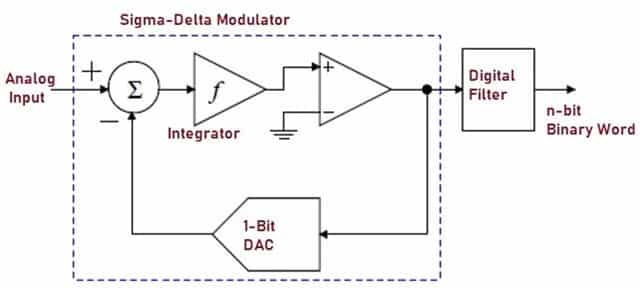Fig. 5 – Block Diagram of Sigma Delta ADC

This type of Analog to Digital Converter incorporates Successive Approximation Algorithm to convert analog input to a digital binary code. Fig. 6 shows the block diagram of Successive Approximation ADC which consists of Comparator, SAR (Successive Approximation Register), Sample and Hold Circuit and DAC.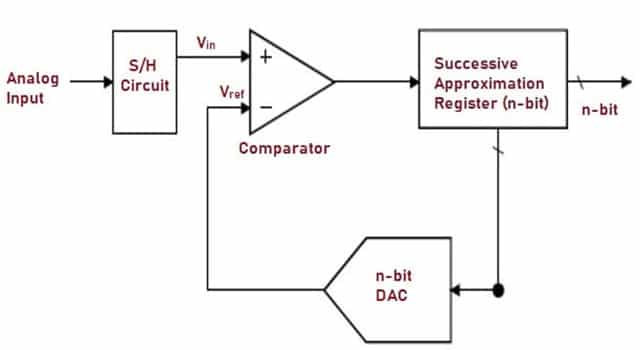Fig. 6 – Block Diagram of Successive Approximation ADC

## How does Analog to Digital Converter Work

To understand the working principle of Analog to Digital Converter, let us consider Fig. 7 below. It is a schematic representation of Successive Approximation Analog to Digital Converter which consists of Comparator, SAR Register, Sample and Hold Circuit (S/H), Clock, n-bit DAC. The SAR Register is incorporated with Binary Search Algorithm.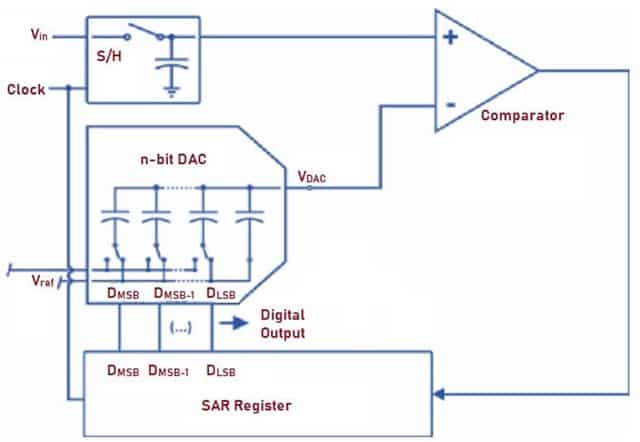Fig. 7 – Schematic Representation of Successive Approximation ADC

The value of the Register is set initially i.e. MSB (Most Significant Bit) is set to “1” and all the other bits are set to “0”. Input Voltage Vin and Clock is applied and Vref is the Reference Voltage of the DAC. When the voltage and clock is applied, the output of DAC (VDAC) becomes half the Reference Voltage (Vref). The input voltage Vin is compared with VDAC by the Comparator.

The output of the Comparator will be set to “1”, if Vin is greater than VDAC. MSB which was set initially remains same i.e. at ‘1’.The output of the Comparator becomes “0”, if the input voltage Vin is less than VDAC. The control logic of the Register will set MSB to ‘0’ and the next bit is set to ‘1’. This process of comparison continues till LSB and n-bit digital output is generated and stored in the Register.

Let us understand the operation of 5- bit ADCs, where Vin = 0.6V and Vref = 1V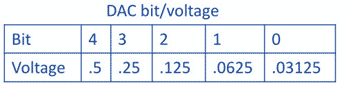There are several applications of Analog to Digital Converter (ADCs) out of which few are listed below:

• They are used in Audio/Video devices.
• They are used in Cell Phones.
• They are used in Digital Multimeters, PLC’s (Programming Logic Controllers).
• They are used in RADAR processing, digital oscilloscopes.
• They are widely used in CMOS Image sensors for mobile applications.
• They are used in Medical Instrumentation and medical imaging.

The advantages of Analog to Digital Converter include:

• Flash ADCs are the fastest compared to the other Analog to Digital Converter.
• Compared to other converters, Sigma Delta ADCs offer high resolution at low-cost.
• Successive Approximation ADCs operate at high speed and are more reliable.
• Sigma-Delta ADCs have higher noise shaping capability and also offer high resolution.
• Pipelined ADCs also offer high resolution at high speed.

• Circuit Complexity increases with the increase in the use of Comparators in Flash ADCs.
• Converting non-periodic signals using Pipeline ADCs can be difficult as it typically runs at a periodic rate.
• Pipeline ADCs are sensitive to board layout.
• Pipeline latency of the input signal occurs in Pipeline ADCs which causes non-linearities in the parameters like Offset and Gain.
• Successive Approximation converters used for higher resolution will be slower.
```Also Read:
What is Digital Voltmeter - How it Works, Types, Applications, Advantages
Digital Communication - Introduction, Basic Components, How Signal Process Works and Advantages
Instrumentation Amplifier - Working Principle, Applications, Advantages
```Laxmi Ashrit
Laxmi is a B.E (Electronics & Communication) and has work experience in RelQ Software as Test Engineer and HP as Technical support executive. She is an author, editor and partner at Electricalfundablog.
RELATED ARTICLES